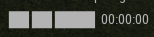# How to make an increasing timer

i have a bodycam and theres a timer on it that should go up the longer you are in the game

its in the hh:mm:ss format
how would i do this?

1 Like

something like this could work

``````local timer = 0

local function FormatTime(sec)
local hours, minutes, seconds

hours = math.floor(sec/3600)
sec = sec%3600

minutes = math.floor(sec/60)
sec = sec%60

seconds = sec

--Use these variables here to update your display
end

while true do
timer += 1
FormateTime(timer)
end
``````
2 Likes

how would i implement those into thisits a textlabel

As taken from this solution here:

You can use this code to format it

``````function Format(Int)
return string.format("%02i", Int)
end

function convertToHMS(Seconds)
local Minutes = (Seconds - Seconds%60)/60
Seconds = Seconds - Minutes*60
local Hours = (Minutes - Minutes%60)/60
Minutes = Minutes - Hours*60
return Format(Hours)..":"..Format(Minutes)..":"..Format(Seconds)
end
``````

And in the `while true do` loop provided by @PoppyandNeivaarecute, make it update the text label by doing `TextLabel.Text = convertToHMS(timer)`

Full code:

``````local TextLabel = path.to.TextLabel

local Timer = 0

function Format(Int)
return string.format("%02i", Int)
end

function convertToHMS(Seconds)
local Minutes = (Seconds - Seconds%60)/60
Seconds = Seconds - Minutes*60
local Hours = (Minutes - Minutes%60)/60
Minutes = Minutes - Hours*60
return Format(Hours)..":"..Format(Minutes)..":"..Format(Seconds)
end

--[[

or use this simplified code taken from another reply to the code above

local function convertToHMS(s)
return string.format("%02i:%02i:%02i", s/60^2, s/60%60, s%60)
end

--]]

Timer += 1
TextLabel.Text = convertToHMS(Timer)
end
``````

In the circumstance the script is counting from the server, you can use a remote event for it to tell the client when the timer is increased

Are those gray boxes textlabels? If so, can I see them in Explorer?

``````local textLabel = script.Parent

local function formatTime(_time)
local hours = math.floor(_time / 3600)
local minutes = math.floor((_time - hours * 3600)/60)
local seconds = math.floor(_time - 3600 * hours - minutes * 60)
return string.format("%02d:%02d:%02d", hours, minutes, seconds)
end

local _time = 0
while true do
You would just need to place this script inside the TextLabel instance, remember to disable the ScreenGui’s `ResetOnSpawn` property if you want the timer to persist across deaths.